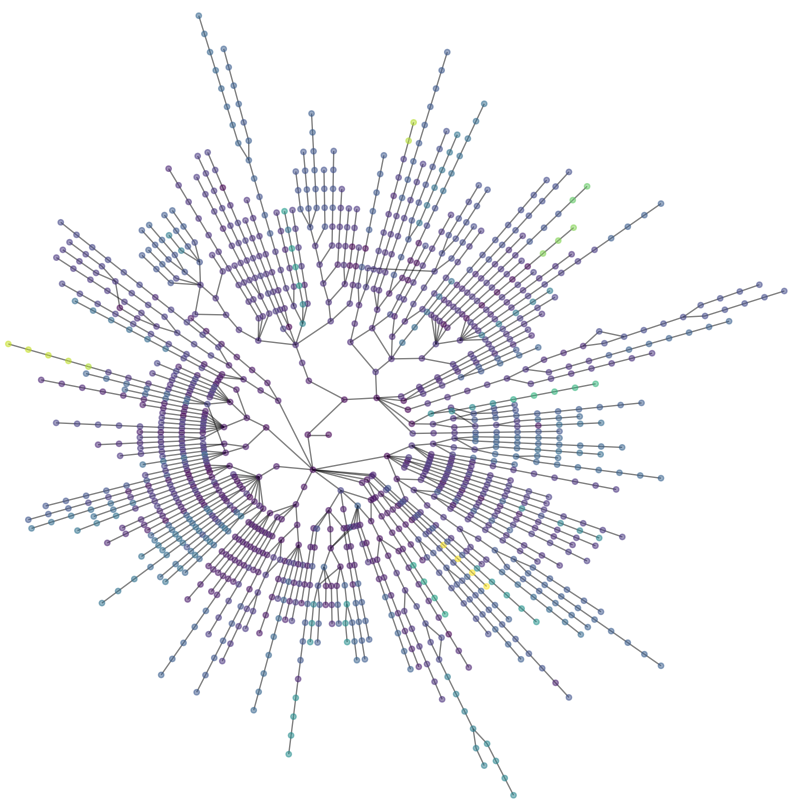# Lanl Routes#

Routes to LANL from 186 sites on the Internet.

The data file can be found at:

This example needs Graphviz and PyGraphviz.```Graph with 1281 nodes and 1296 edges
1 connected components
```

```import matplotlib.pyplot as plt
import networkx as nx

def lanl_graph():
"""Return the lanl internet view graph from lanl.edges"""
try:
fh = open("lanl_routes.edgelist")
except OSError:
raise

G = nx.Graph()

time = {}
time = 0  # assign 0 to center node

# get largest component and assign ping times to G0time dictionary
Gcc = sorted(nx.connected_components(G), key=len, reverse=True)
G0 = G.subgraph(Gcc)
G0.rtt = {}
for n in G0:
G0.rtt[n] = time[n]

return G0

G = lanl_graph()

print(G)
print(nx.number_connected_components(G), "connected components")

plt.figure(figsize=(8, 8))
# use graphviz to find radial layout
pos = nx.nx_agraph.graphviz_layout(G, prog="twopi", root=0)
# draw nodes, coloring by rtt ping time
options = {"with_labels": False, "alpha": 0.5, "node_size": 15}
nx.draw(G, pos, node_color=[G.rtt[v] for v in G], **options)